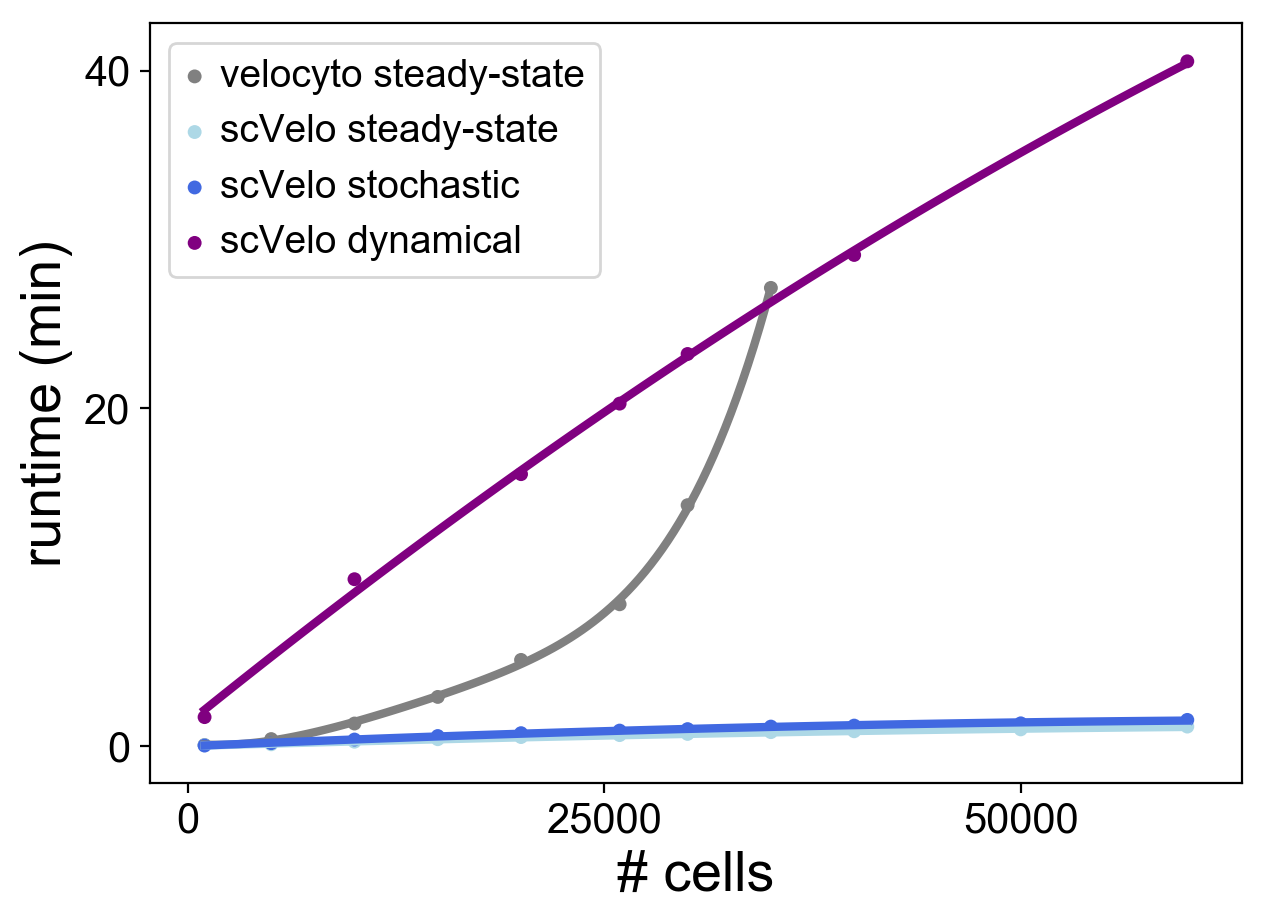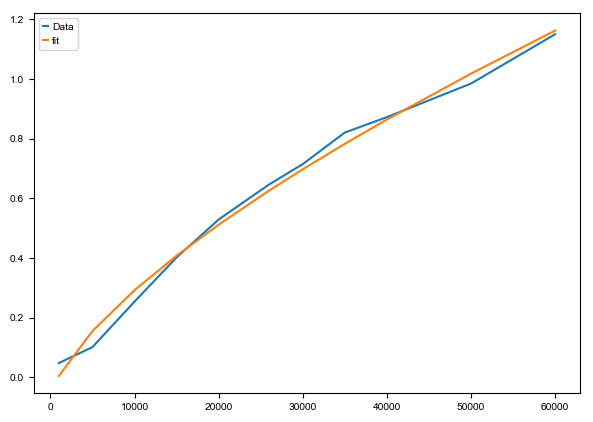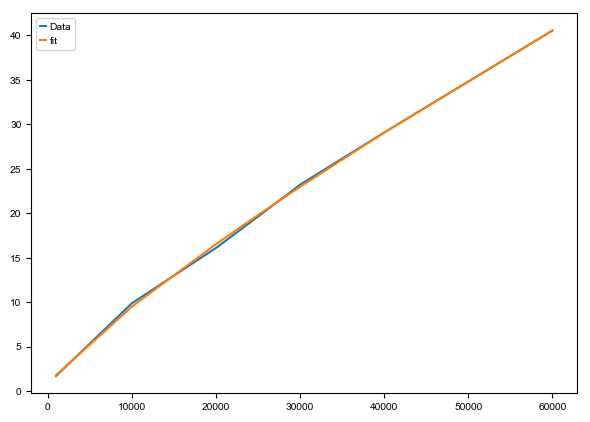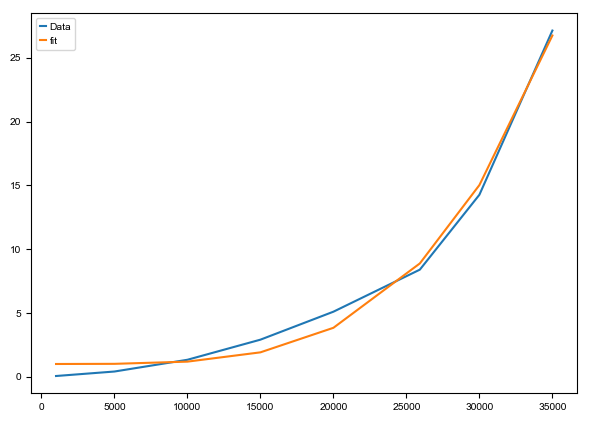In :
import matplotlib.pyplot as pl
import numpy as np
import pandas as pd
import scanpy as sc
from time import time

import scvelo as scv
scv.logging.print_versions()

scvelo==0.1.24  scanpy==1.4.5.dev136+gd8f32c0  anndata==0.6.22.post2.dev82+g6310e3e  loompy==3.0.0  numpy==1.17.2  scipy==1.3.1  matplotlib==3.0.3  sklearn==0.21.3  pandas==0.25.1

In :
scv.settings.set_figure_params(style='scvelo')
scv.settings.verbosity = 0

In :
def run_scv(adata, mode='steady_state'):
scv.pp.filter_and_normalize(adata, min_counts=20, min_counts_u=10, n_top_genes=1000)
scv.pp.moments(adata, method='hnsw', n_pcs=30, n_neighbors=30)

if mode is 'dynamical':
else:
try:
except:
print("neighbor graph corrupted")

def run_vcy(vlm):
vlm.score_detection_levels(min_expr_counts=20, min_expr_counts_U=10)
vlm.filter_genes(by_detection_levels=True)

if vlm.S.shape > 1000:
vlm.score_cv_vs_mean(1000)
vlm.filter_genes(by_cv_vs_mean=True)

vlm._normalize_S(relative_size=vlm.initial_cell_size, target_size=np.median(vlm.initial_cell_size))
vlm._normalize_U(relative_size=vlm.initial_Ucell_size, target_size=np.median(vlm.initial_Ucell_size))

vlm.S_norm = np.log1p(vlm.S_sz)

vlm.perform_PCA(n_components=30)
vlm.knn_imputation(n_pca_dims=30, k=30)
vlm.normalize_median()

vlm.fit_gammas()
vlm.filter_genes_good_fit()

vlm.predict_U()
vlm.calculate_velocity()
vlm.calculate_shift()
vlm.extrapolate_cell_at_t()

vlm.estimate_transition_prob()
vlm.calculate_embedding_shift()
vlm.calculate_grid_arrows()

In :
adata_ref = scv.read('data/pancreas/endocrinogenesis.h5ad')

In :
test = False

In :
#scvelo's steady-state and stochastic model
np.random.seed(42)
n_cells = np.array([1e3, 5e3, 10e3, 15e3, 20e3, 25919, 30e3, 35e3, 40e3, 50e3, 60e3], dtype=int)
if test: n_cells = np.array([.5e3, 1e3], dtype=int)
scv_stoch_time, scv_time = [], []

for n in n_cells:
indices = np.random.choice(adata_ref.n_obs, n)

t_init = time()
scv_time.extend([(time() - t_init) / 60])

t_init = time()
scv_stoch_time.extend([(time() - t_init) / 60])

np.save('data/runtime/scv_time' + str(n), scv_time[-1])
np.save('data/runtime/scv_stoch_time' + str(n), scv_stoch_time[-1])

scv_time = np.array(scv_time)
scv_stoch_time = np.array(scv_stoch_time)

np.save('data/runtime/n_cells', n_cells)
np.save('data/runtime/scv_time', scv_time)
np.save('data/runtime/scv_stoch_time', scv_stoch_time)

In :
#velocyto's steady-state model
np.random.seed(42)
n_cells = np.array([1e3, 5e3, 10e3, 15e3, 20e3, 25919, 30e3, 35e3, 40e3, 50e3, 60e3], dtype=int)  # run out of 64GB RAM at 40k cells
if test: n_cells = np.array([.5e3, 1e3], dtype=int)
vcy_time = []

for n in n_cells:
indices = np.random.choice(adata_ref.n_obs, n)

vlm = scv.utils.convert_to_loom(adata_run, basis='umap')

t_init = time()
run_vcy(vlm)
vcy_time.extend([(time() - t_init) / 60])

np.save('data/runtime/vcy_time' + str(n), vcy_time[-1])

vcy_time = np.array(vcy_time)
np.save('data/runtime/vcy_time', vcy_time)

WARNING:root:object does not have the attribute small_U_pop, so all the unspliced will be normalized by relative size, this might cause the overinflation the unspliced counts of cells where only few unspliced molecules were detected
WARNING:root:Nans encountered in corrcoef and corrected to 1s. If not identical cells were present it is probably a small isolated cluster converging after imputation.
WARNING:root:Nans encountered in corrcoef_random and corrected to 1s. If not identical cells were present it is probably a small isolated cluster converging after imputation.
WARNING:root:object does not have the attribute small_U_pop, so all the unspliced will be normalized by relative size, this might cause the overinflation the unspliced counts of cells where only few unspliced molecules were detected
WARNING:root:Nans encountered in corrcoef and corrected to 1s. If not identical cells were present it is probably a small isolated cluster converging after imputation.
WARNING:root:Nans encountered in corrcoef_random and corrected to 1s. If not identical cells were present it is probably a small isolated cluster converging after imputation.
WARNING:root:object does not have the attribute small_U_pop, so all the unspliced will be normalized by relative size, this might cause the overinflation the unspliced counts of cells where only few unspliced molecules were detected
WARNING:root:Nans encountered in corrcoef and corrected to 1s. If not identical cells were present it is probably a small isolated cluster converging after imputation.
WARNING:root:Nans encountered in corrcoef_random and corrected to 1s. If not identical cells were present it is probably a small isolated cluster converging after imputation.
WARNING:root:object does not have the attribute small_U_pop, so all the unspliced will be normalized by relative size, this might cause the overinflation the unspliced counts of cells where only few unspliced molecules were detected
WARNING:root:Nans encountered in corrcoef and corrected to 1s. If not identical cells were present it is probably a small isolated cluster converging after imputation.
WARNING:root:Nans encountered in corrcoef_random and corrected to 1s. If not identical cells were present it is probably a small isolated cluster converging after imputation.
WARNING:root:object does not have the attribute small_U_pop, so all the unspliced will be normalized by relative size, this might cause the overinflation the unspliced counts of cells where only few unspliced molecules were detected
WARNING:root:Nans encountered in corrcoef and corrected to 1s. If not identical cells were present it is probably a small isolated cluster converging after imputation.
WARNING:root:Nans encountered in corrcoef_random and corrected to 1s. If not identical cells were present it is probably a small isolated cluster converging after imputation.
WARNING:root:object does not have the attribute small_U_pop, so all the unspliced will be normalized by relative size, this might cause the overinflation the unspliced counts of cells where only few unspliced molecules were detected
WARNING:root:Nans encountered in corrcoef and corrected to 1s. If not identical cells were present it is probably a small isolated cluster converging after imputation.
WARNING:root:Nans encountered in corrcoef_random and corrected to 1s. If not identical cells were present it is probably a small isolated cluster converging after imputation.
WARNING:root:object does not have the attribute small_U_pop, so all the unspliced will be normalized by relative size, this might cause the overinflation the unspliced counts of cells where only few unspliced molecules were detected
WARNING:root:Nans encountered in corrcoef and corrected to 1s. If not identical cells were present it is probably a small isolated cluster converging after imputation.
WARNING:root:Nans encountered in corrcoef_random and corrected to 1s. If not identical cells were present it is probably a small isolated cluster converging after imputation.
WARNING:root:object does not have the attribute small_U_pop, so all the unspliced will be normalized by relative size, this might cause the overinflation the unspliced counts of cells where only few unspliced molecules were detected
WARNING:root:Nans encountered in corrcoef and corrected to 1s. If not identical cells were present it is probably a small isolated cluster converging after imputation.
WARNING:root:Nans encountered in corrcoef_random and corrected to 1s. If not identical cells were present it is probably a small isolated cluster converging after imputation.
WARNING:root:object does not have the attribute small_U_pop, so all the unspliced will be normalized by relative size, this might cause the overinflation the unspliced counts of cells where only few unspliced molecules were detected
WARNING:root:Nans encountered in corrcoef and corrected to 1s. If not identical cells were present it is probably a small isolated cluster converging after imputation.
WARNING:root:Nans encountered in corrcoef_random and corrected to 1s. If not identical cells were present it is probably a small isolated cluster converging after imputation.

---------------------------------------------------------------------------
KeyboardInterrupt                         Traceback (most recent call last)
<ipython-input-7-17705123488f> in <module>
17
18     t_init = time()
---> 19     run_vcy(vlm)
20     vcy_time.extend([(time() - t_init) / 60])
21

<ipython-input-3-90869d0351a5> in run_vcy(vlm)
41
42     vlm.estimate_transition_prob()
---> 43     vlm.calculate_embedding_shift()
44     vlm.calculate_grid_arrows()

~/velocyto.py/velocyto/analysis.py in calculate_embedding_shift(self, sigma_corr, expression_scaling, scaling_penalty)
1702                 self.transition_prob_random /= self.transition_prob_random.sum(1)[:, None]
1703
-> 1704             unitary_vectors = self.embedding.T[:, None, :] - self.embedding.T[:, :, None]  # shape (2,ncells,ncells)
1705             with np.errstate(divide='ignore', invalid='ignore'):
1706                 unitary_vectors /= np.linalg.norm(unitary_vectors, ord=2, axis=0)  # divide by L2

KeyboardInterrupt: 
In :
vcy_time = np.array(vcy_time)
np.save('data/runtime/vcy_time', vcy_time)

In :
#scvelo's dynamical model
np.random.seed(42)
n_dyn_cells = np.array([1e3, 10e3, 20e3, 25919, 30e3, 40e3, 60e3], dtype=int)
if test: n_dyn_cells = np.array([.5e3, 1e3], dtype=int)
scv_dyn_time = []

for n in n_dyn_cells:
indices = np.random.choice(adata_ref.n_obs, n)

t_init = time()
scv_dyn_time.extend([(time() - t_init) / 60])

np.save('data/runtime/scv_dyn_time' + str(n), scv_dyn_time[-1])

scv_dyn_time = np.array(scv_dyn_time)
np.save('data/runtime/n_dyn_cells', n_dyn_cells)
np.save('data/runtime/scv_dyn_time', scv_dyn_time)

In :
#scvelo's steady-state and stochastic model second run with large cell numbers
np.random.seed(2020)
n_large_cells = np.array([100e3, 150e3, 200e3, 250e3, 300e3], dtype=int)
if test: n_large_cells = np.array([.5e3, 1e3], dtype=int)
scv_stoch_large_time, scv_large_time = [], []

for n in n_large_cells:
indices = np.random.choice(adata_ref.n_obs, n)

t_init = time()
scv_large_time.extend([(time() - t_init) / 60])

t_init = time()
scv_stoch_large_time.extend([(time() - t_init) / 60])

np.save('data/runtime/scv_large_time' + str(n), scv_large_time[-1])
np.save('data/runtime/scv_stoch_large_time' + str(n), scv_stoch_large_time[-1])

scv_large_time = np.array(scv_large_time)
scv_stoch_large_time = np.array(scv_stoch_large_time)
np.save('data/runtime/n_large_cells', n_large_cells)
np.save('data/runtime/scv_large_time', scv_large_time)
np.save('data/runtime/scv_stoch_large_time', scv_stoch_large_time)

In :
n_cells = scv.load('data/runtime/n_cells.npy')


In :
vcy_time_ext = np.hstack([vcy_time, np.ones(len(n_cells) - len(vcy_time))*np.nan])
pd.DataFrame(np.vstack([n_cells, scv_time, scv_stoch_time, vcy_time_ext]).T.round(2),
columns=['n_cells', 'scvelo steady-state', 'scvelo stochastic', 'velocyto steady-state'])

Out:
n_cells scvelo steady-state scvelo stochastic velocyto steady-state
0 1000.0 0.05 0.02 0.06
1 5000.0 0.10 0.17 0.41
2 10000.0 0.25 0.38 1.33
3 15000.0 0.40 0.59 2.91
4 20000.0 0.53 0.76 5.10
5 25919.0 0.64 0.91 8.39
6 30000.0 0.71 1.00 14.26
7 35000.0 0.82 1.15 27.13
8 40000.0 0.87 1.21 NaN
9 50000.0 0.98 1.34 NaN
10 60000.0 1.15 1.54 NaN
In :
kwargs = {'show': False, 'fontsize': 20, 'size': 100, 'linewidth': 3}
'scVelo stochastic', 'scVelo dynamical']

ax = scv.pl.scatter(x=n_cells[:len(vcy_time)], y=vcy_time, **kwargs, show_polyfit=5, label=labels)
ax = scv.pl.scatter(x=n_cells, y=scv_time, color='lightblue', ax=ax, **kwargs, show_polyfit=True, label=labels)
ax = scv.pl.scatter(x=n_cells, y=scv_stoch_time, ax=ax, color='royalblue', **kwargs, show_polyfit=True, label=labels)
ax = scv.pl.scatter(x=n_dyn_cells, y=scv_dyn_time, ax=ax, color='purple', **kwargs, show_polyfit=True, label=labels)

pl.legend(fontsize=14)
pl.xlabel('# cells', fontsize=20);
pl.ylabel('runtime (min)', fontsize=20);In :
from scipy.optimize import curve_fit

def expo(x, a, b, c):
o = 1 if np.isscalar(x) else np.ones(len(x))
return a * x ** b + c * o

def fit(x, y, n_extrapol=None):
def do_fit(function, x, y):
popt, pcov = curve_fit(eval(function), x, y, maxfev=10000)
print('complexity: ', popt)
#print('Standard error fit: ', np.sqrt(np.diag(pcov)))
return popt, pcov

ax=pl.figure(dpi=50).gca()
x = np.array(x)
ax.plot(x, y)

popt, pcov = do_fit('expo', x, y)
ax.plot(x, expo(x, popt, popt, popt))
pl.legend(['Data', 'fit'])

pl.show()
if n_extrapol is not None:
return expo(n_extrapol, popt, popt, popt)

fit(n_cells, scv_time)
fit(n_dyn_cells, scv_dyn_time)
fit(n_cells[:len(vcy_time)], vcy_time)

complexity:  0.6825109901178577complexity:  0.8324485537356342complexity:  3.9414349162779394